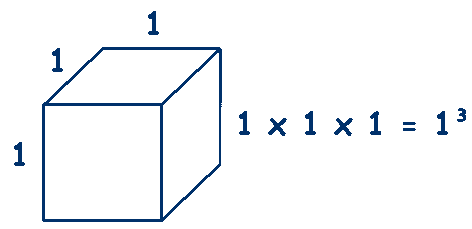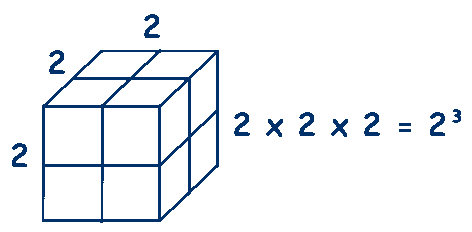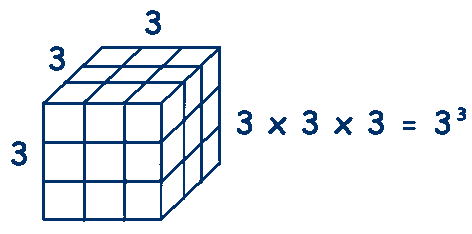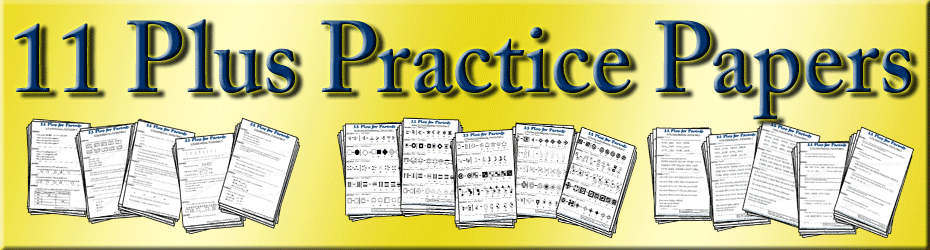# Cube Numbers

## A Cube Number is a number that is multiplied by itself and then multiplied by itself again.

### They are called cube numbers because these numbers do form cubes.The smallest cube we can make with whole numbers (integers) is 1 unit by 1 unit by 1 unit

## 1 x 1 x 1 = 1³ = 1

### so 1 is the first cube number

The next cube we can make with whole numbers (integers) is 2 units by 2 units by 2 units

## 2 x 2 x 2 = 2³ = 8

### so 8 is the next cube number

The next cube we can make with whole numbers (integers) is 3 units by 3 units by 3 units

## 3 x 3 x 3 = 3³ = 27

### so 27 is the next cube number

I usually work through a few more numbers up to 10, it doesn't take long after they get the idea and you end up with the first ten cube numbers.

## 1, 8, 27, 64, 125, 216, 343, 512, 729 and 1000

It is worth remembering the first five of these numbers as they will come up again.

It is a good idea to be able to visualise how a cube is made up - if you have a rubik's cube, that is ideal for showing 3³ or you could use Oxo cubes to build cubes of different sizes.

It may be worth introducing the fact that using this small number placed after and above, to show that the number needs to be multiplied by itself a number of times is known as 'Index form' and that the small digit is known as the 'index number' or 'power'.

The index number tells you that the number appears that many times in multiplying.

When the index number is 2, as in 5² it means 5 x 5 and can be called either
'five squared' or 'five to the power of two'.

When the index number is 3, as in 5³ it means 5 x 5 x 5 and can be called either
'five cubed' or 'five to the power of three'.